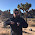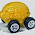Thursday, May 28, 2015

Resolving the Cambridge capital controvery with abstract algebra

The title is a bit of a joke, and for the controversy see here. Looking into the Solow model bumps you into the question of what "capital" (K) is, and that met with the titular controversy awhile back where Cambridge, MA said you could add up different stuff in a sensible way while Cambridge, UK said you couldn't.

The information equilibrium (IE) model calls the argument for the UK (and Joan Robinson), but allows (at least) two possibilities for definitions of capital that are sensible. These sensible definitions weren't advocated by Solow/Samuelson at MIT, hence why I say that the UK won the debate: you can't just add stuff up and get a sensible answer.

First, two quick "proofs". I already showed IE is an equivalence relation (you can use it to define a set of things in IE with some economic aggregate), but I need a bit more: IE is a group under multiplication.

If $A \rightleftarrows K$ (with IT index $a$), then $A^{x} \rightleftarrows K$ (with IT index $a x$) because:

$$\frac{d}{dK} A^{x} = x A^{x - 1} \frac{dA}{dK} = a x \frac{A^{x}}{K}$$

(I show this because it applies for real exponents rather than just natural numbers and that might be important for some reason in the future; for natural numbers $x$ the following result would suffice.)

If $A \rightleftarrows K$ and $B \rightleftarrows K$ (with IT indices $a$ and $b$), then $A B \rightleftarrows K$ (with IT index $a + b$) because:

$$\frac{d}{dK} AB = \frac{dA}{dK} B + A \frac{dB}{dK} = (a + b) \frac{AB}{K}$$

So we have the set of all things that are in IE with $K$, and the product of any two of those things is another thing in the set — therefore it's a group. It is not, however, a ring — the set isn't closed under addition:

$$\frac{d}{dK} (A + B) = \frac{dA}{dK} + \frac{dB}{dK} = \frac{a A + b B}{K}$$

so $A + B$ is not in IE with $K$ unless $a = b$.

This basically was Joan Robinson's point — unless $A$ and $B$ are the same thing, you're comparing apples and oranges. Money doesn't help us either and if you introduce it, the relative prices of the capital goods become important **.

Sensible definitions of capital

Of course, this points to the first sensible solution to the capital controversy: instead of adding up capital items, use the geometric mean. Using the two results above, you can show that if $A \rightleftarrows K$, $B \rightleftarrows K$, $C \rightleftarrows K$, etc, then

$$(A B C \; ... \;)^{1/n} \rightleftarrows K$$

The geometric mean is also the only sensible mean for capital goods measured either as indices or in terms of money.

The second sensible solution to the controversy is a partition function approach (as I've done here) where we simply define capital to be the expected value of the capital operator, which is just the sum of the individual capital goods operators:

$$\langle K \rangle \equiv \langle A + B + C + \; ... \; \rangle$$

In that sense, "capital" would be more like NGDP than, say, a stock index.

...

** Update 29 May 2015

I thought I'd add in the details of the sentence I marked with ** above. We assume two goods markets (information equilibrium conditions) $p_{a} : N \rightleftarrows A$ and $p_{b} : N \rightleftarrows B$ where $N$ is aggregate demand/nominal output measured in money and the $p_{i}$ are prices. That gives us:

$$k_{a} p_{a} A = N \; \text{and} \; k_{b} p_{b} B = N$$

Substituting into the formula above

$$\frac{d}{dK} (A + B) = \frac{a A + b B}{K} = \left( \frac{a}{k_{a} p_{a}} + \frac{b}{k_{b} p_{b}} \right) \; \frac{N}{K}$$

which basically shows that $K$ is in information equilibrium with aggregate demand. Note the appearance of the prices in the information transfer index.

...

Update 14 October 2018

Changed the old notation $A \rightarrow B$ to the better notation for an information equilibrium relationship: $A \rightleftarrows B$. Added "measured in money" to 29 May 2015 update.

1.Don't understand a word of your post but loved the title !

1.Thanks, rob. I thought it was pretty ridiculous.

2.This strikes me as good. Now just show that at equilibrium all rbc models are in information equilibrium and now we finally know how to measure capital to being them to test with the gmm

1.Hi LAL, not sure what that last clause is -- I got the acronym for generalized method of moments.

Actually, I think GMM might be similar to information equilibrium. In both cases, instead of something being exactly equal in the traditional sense, you take it to have some slightly less stringent definition of equality. For IE, that is the information content of the time series is equal, for GMM it is the moments of the time series.

I think it might even be a hierarchy like this:

equal > proportional > IE > GMM

3.This is the most neat thing I have seen on this blog. Bookmarked.

1.Cheers, MSL.

4.That's very nice.

1.5.I understood some of those words.

1.I thought it was a pretty silly way to address the CCC. One sentence summary: Joan Robinson was right about capital.

Comments are welcome. Please see the Moderation and comment policy.

Also, try to avoid the use of dollar signs as they interfere with my setup of mathjax. I left it set up that way because I think this is funny for an economics blog. You can use € or £ instead.